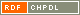# Show document

Title: A note on domination and independence-domination numbers of graphs Milanič, Martin (Author)http://amc.imfm.si/index.php/amc/article/view/282 English Not categorized 1.08 - Published Scientific Conference Contribution IAM - Andrej Marušič Institute Vizing's conjecture is true for graphs ▫$G$▫ satisfying ▫$\gamma^i(G) = \gamma(G)$▫, where ▫$\gamma(G)$▫ is the domination number of a graph ▫$G$▫ and ▫$\gamma^i(G)$▫ is the independence-domination number of ▫$G$▫, that is, the maximum, over all independent sets ▫$I$▫ in ▫$G$▫, of the minimum number of vertices needed to dominate ▫$I$▫. The equality ▫$\gamma^i(G) = \gamma(G)$▫ is known to hold for all chordal graphs and for chordless cycles of length ▫$0 \pmod{3}$▫. We prove some results related to graphs for which the above equality holds. More specifically, we show that the problems of determining whether ▫$\gamma^i(G) = \gamma(G) = 2$▫ and of verifying whether ▫$\gamma^i(G) \ge 2$▫ are NP-complete, even if ▫$G$▫ is weakly chordal. We also initiate the study of the equality ▫$\gamma^i = \gamma$▫ in the context of hereditary graph classes and exhibit two infinite families of graphs for which ▫$\gamma^i < \gamma$▫. Vizing's conjecture, domination number, independence-domination number, weakly chordal graph, NP-completeness, hereditary graph class, IDD-perfect graph 2013 str. 89-97 Vol. 6, no. 1 1855-3966 519.17 10244237641466 77Document is not linked to any category.

Average score: (0 votes) Voting is allowed only to logged in users.Hover the mouse pointer over a document title to show the abstract or click on the title to get all document metadata.